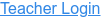### Topics

• Ratios
• Proportional Reasoning
• Double Number Line
• Unit Rate

### Description

Discover the unit rate while shopping for fruits, vegetables, and candy. Construct a double number line and look for patterns. Challenge yourself on the race track as you compare cars with different rates!

### Sample Learning Goals

• Interpret ratios of mixed units (e.g. \$/lb)
• Define the unit rate and determine a method to calculate it
• Predict how changing the numerator or denominator of a rate will affect the unit rate
• Use the double number line to reason about rates and solve real-world problems
• Develop strategies to use the unit rate to solve problems
• Compare unit rates between two simultaneous situations
Version 1.0.5